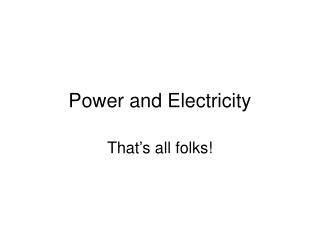Download PresentationPower and ElectricityPower and Electricity - PowerPoint PPT Presentation

Download PresentationPower and Electricity
An Image/Link below is provided (as is) to download presentation

Download Policy: Content on the Website is provided to you AS IS for your information and personal use and may not be sold / licensed / shared on other websites without getting consent from its author. While downloading, if for some reason you are not able to download a presentation, the publisher may have deleted the file from their server.

- - - - - - - - - - - - - - - - - - - - - - - - - - - E N D - - - - - - - - - - - - - - - - - - - - - - - - - - -
Presentation Transcript

1. Power and Electricity That’s all folks!

2. Power • Power is how quickly work is done • Measured in watts (W) and kilowatts (kW) Power (W) = Work (J) / time (s)

3. Power can be Electrical or Mechanical • Electrical when measuring… • rate of electrical energy used in a microwave • Mechanical when measuring… • rate of mechanical energy used running up stairs

4. We Buy Energy • To calculate power bill 1. Find power rating of appliance (in kW) 2. Multiply by time used per month (in hours) to get energy used (in kWhr) 3. Multiply by cost per kWhr • Calculate the cost for using a 500W (0.5 kW) computer for 13 hours at \$.08/kWhr.

5. Electricity • Flow of charge through a __________ • Circuit must be ___________ • Current flows from ______ electrical potential (or high voltage) to _____electrical potential (low voltage) circuit closed high low

6. Voltage (V) • Electrical Push • Potential Difference • Supplied by battery (DC) or power plant (AC) • Measured in volts (V)

7. Resistance (R) • How hard does an object make it for current to flow? • Measured in ohms (Ω) Current (I) • How quickly charge flows • Measured in amps or amperes (A)

8. Ohm’s Law • More voltage means more current • More resistance means less current

9. Series Circuit • Total voltage is used up by the resistors on the one pathway (voltage is divided among resistors) • Current is the same everywhere in circuit • Find total resistance by adding all resistors

10. Parallel Circuit • Each branch gets total voltage • Each branch has its own resistance • Calculate current in each branch and add up to find total current • Find total R by dividing V by total I

11. One light out, all out More resistors, current goes down, and lights get dim One light out, others stay on More resistors (on new branches), total current goes up More current flowing means more friction in wires, more heat generated, possible fire hazard Series vs. Parallel